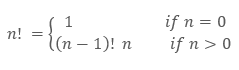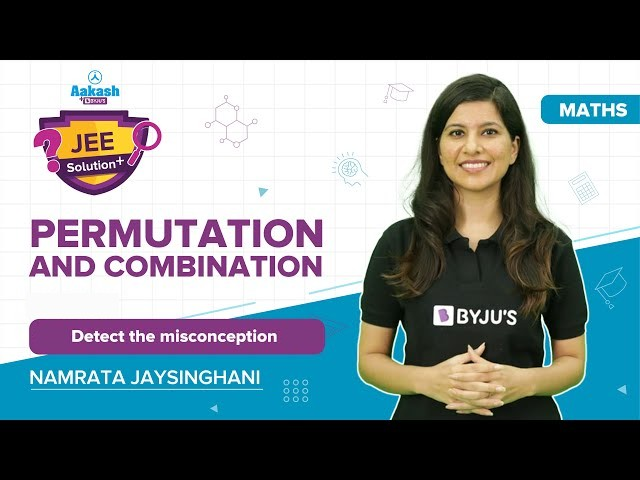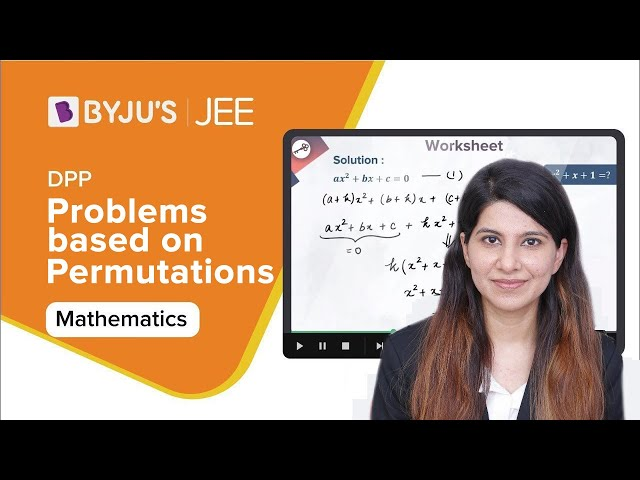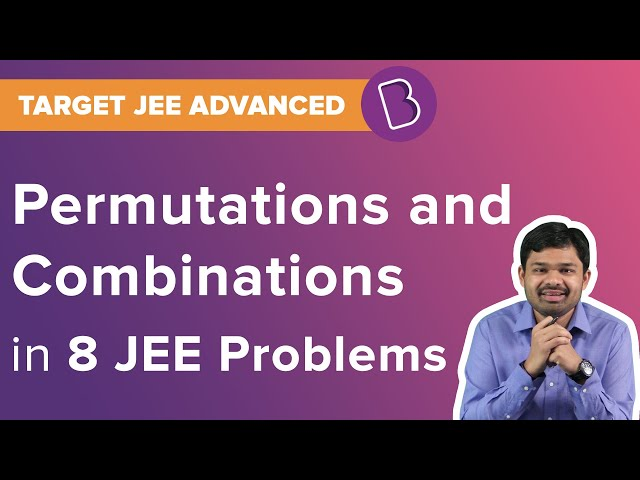# Permutation And Combination Solved Problems

Introduction To Permutation And Combination

The word permutations and combinations is concerned with finding the number of different ways of arranging and selecting objects out of a given number of objects, without actually listing them. When the order does not matter, it is a combination. When the order matters, it is a permutation. This article explains the definition and gives solved problems on permutations and combinations.

A permutation is the arrangement of things in an ordered way without repetition. It follows from the principle of counting. Factorial can be defined as the set of positive integers that are less than or equal to “n”. The mathematical notation of factorial is as follows:where n is a positive integer.

N factorial [N!] represents the number of ways the set can be permuted.

N! = N × (N – 1) × (N – 2) × . . . × 1

The general formula for permutation is given by

nPr = n! / (n – r)!

where n represents the number of objects and r the number of objects taken at that time.

For example, say you are required to arrange English, Hindi, Mathematics, Science and Sanskrit textbooks on a shelf. In how many possible ways can it be done? Here 5 books need to be arranged. The number of permutations of n objects is n! = n (n – 1) (n – 2) … 2 . 1

Here n = 5. So, the number of permutations is 5! = 5 * 4 * 3 * 2 * 1 = 120. Hence, 5 books can be arranged in 120 ways.

Combination is the number of ways an object can be selected from a group. The order is not taken into consideration here and the objects can be repeated. The synonyms of combination can be selection or collection. The general formula for combination is given by

nCr = n!/ r!(n – r)!

where n represents the number of objects and r the number of objects taken at that time. For instance, the selection of different subject teachers from a group of 10 teachers in random order.

## Solved Problems On Permutation And Combination for IIT JEE

Example 1: Total number of six-digit numbers that can be formed having the property that every succeeding digit is greater than the preceding digit is equal to _________.

Solution:

x1 < x2 < x3 < x4 <x5 < x6, when the numbers are x1 x2 x3 x4 x5 x6 and clearly no digit can be zero. Also, all the digits are distinct. So. Let us first select six digits from the list of digits 1, 2, 3, 4, 5, 6, 7, 8, 9, which can be done in 9C6 ways. After selecting these digits they can be put only in one order. Thus, a total number of such numbers is 9C6 × 1 = 9C3 = 84.

Example 2: Find the number of words of four letters containing an equal number of vowels and consonants, where repetition is allowed.

Solution:

Let us first select two places for vowel, which can be selected from 4 places in 4C2 ways. Now this places can be filled by vowels in 5 × 5 =25 ways as repetition is allowed. The remaining two places can be filled by consonants in 21 × 21 ways. Then the total number of words is 4C2 × 25 × 212 = 150 × 212.

Example 3: A man has three friends. The number of ways he can invite one friend every day for dinner on six successive nights so that no friend is invited more than three times is ________.

Solution:

Let x, y, z be the friends and a, b, c denote the case when x is invited, we have the following possibilities: (a, b, c) = (1, 2, 3) or (3, 3, 0) or (2, 2, 2) [grouping of 6 days of week].

Hence, the total number of ways is (6! / [1! 2! 3!]) * 3! + (6! / [3! 3! 2!]) * 3! + (6! / [2! 2! 2!]) * 3! = 510

Example 4: The number of ways of choosing a committee of two women and three men from five women and six men. If Mr A refuses to serve on the committee if Mr B is a member and Mr B can only serve if Miss C is the member of the committee is ____________.

Solution:

(i) Miss C is taken

[a] B included ⇒ A excluded ⇒ 4C1 × 4C2 = 24

[b] B excluded ⇒ 4C1 × 5C3 = 40

Miss C is not taken

⇒ B does not come ⇒4C2 × 5C3 = 60

⇒ Total = 124

Alternate method:

Case I:

Mr B is present

⇒A is excluded and C included

Hence, the number of ways is 4C2 4C1 = 24.

Case II:

Mr B is absent

⇒No constraint

Hence, the number of ways is 5C3 5C2 = 100.

∴Total = 124

Example 5: Find the sum of all the numbers of four different digits that can be made by using the digits 0, 1, 2, and 3.

Solution:

The number of numbers with 0 in the units place is 3! = 6. The number of numbers with 1 or 2 or 3 in the units place is 6 × 0 × +4 × 1 + 4 × 2 + 4 × 3 = 24. Similarly, for the tens and hundred places, the number of numbers with 1 or 2 in the thousands place is 3! Therefore, the sum of the digits in the thousands place is 6 × 1 + 6 × 2 + 6 × 3 = 36. Hence, the required sum is 36 × 1000 + 24 × 100 + 24 × 10 + 24 = 38664.

Example 6: The number of different words that can be formed out of the letters of the word ‘MORADABAD’ taken four at a time is

A) 500

B) 600

C) 620

D) 626

Solution:

In MORADABAD, we have 6 different types of letters $3{{A}^{s}},2{{D}^{s}}$ and the rest four different. We have to form words of 4 letters.

(i) All different

$^{6}{{P}_{4}}=6\times 5\times 4\times 3=360$

(ii) Two different two alike

$^{2}{{C}_{1}}{{\times }^{5}}{{C}_{2}}\times \frac{4\ !}{2\,\,!}=240$

(iii) 3 alike 1 different

$^{1}{{C}_{1}}{{\times }^{5}}{{C}_{1}}\times \frac{4\ !}{3\ !}=20$

(iv) 2 alike of one type and 2 alike of other type

$^{2}{{C}_{2}}\times \frac{4\ !}{2\ !\ 2\ !}=6$

Therefore total number of words = 360 + 240 + 20 + 6 = 626.

Example 7: How many numbers between 5000 and 10,000 can be formed using the digits 1, 2, 3, 4, 5, 6, 7, 8, 9 each digit appearing not more than once in each number

$A) 5{{\times }^{8}}{{P}_{3}}\\ B) 5{{\times }^{8}}{{C}_{3}}\\ C) 5\ !\ {{\times }^{8}}{{P}_{3}}\\ D) 5\ !\ {{\times }^{8}}{{C}_{3}}$

Solution:

A number between 5000 and 10,000 can have any of the digits 5, 6, 7, 8, 9 at thousand’s place. So thousand’s place can be filled in 5 ways. Remaining 3 places can be filled by the remaining 8 digits in $^{8}{{P}_{3}}$ ways. Hence required number = $5{{\times }^{8}}{{P}_{3}}.$

Example 8: There are (n + 1) white and (n + 1) black balls each set numbered 1 to n + 1. The number of ways in which the balls can be arranged in a row so that the adjacent balls are of different colours is

A) (2n + 2) !

B) (2n + 2) ! × 2

C) (n + 1) ! × 2

D) $2{{\{(n+1)\ !\}}^{2}}$

Solution:

Since the balls are to be arranged in a row so that the adjacent balls are of different colours, therefore we can begin with a white ball or a black ball. If we begin with a white ball, we find that (n + 1) white balls numbered 1 to (n + 1) can be arranged in a row in (n + 1) ! ways. Now (n + 2) places are created between n + 1 white balls which can be filled by (n + 1) black balls in (n + 1) ! ways. So the total number of arrangements in which adjacent balls are of different colours and first ball is a white ball is

$(n+1)\ !\ \times (n+1)\ !\ ={{[(n+1)\ !]}^{2}}$.

But we can begin with a black ball also. Hence the required number of arrangements is

$2{{[(n+1)\ !]}^{2}}$

Example 9: A car will hold 2 in the front seat and 1 in the rear seat. If among 6 persons 2 can drive, then the number of ways in which the car can be filled is

A) 10

B) 20

C) 30

D) None of these

Solution:

Since 2 persons can drive the car, therefore we have to select 1 from these two. This can be done in $^{2}{{C}_{1}}$ ways.

Now we have the remaining 5 persons.

So another set of 5 persons can sit in the front seat in 5C1 ways.

Now the back seat can be filled by the remaining 4 persons in 4C1 ways.

Therefore, total number of ways = 2C1 x 5C1 x 4C1 = 2x5x4 = 40

Example 10: A committee of 3 persons is to be formed from a group of 2 men and 3 women. In how many ways this can be done? How many of these committees would contain 1 man and 2 women.

Solution:

There will be as many sets as there are combinations of 5 different persons taken 3 at a time. So the required number of ways = 5C3 = 5!/(3! 2 !)

= (4×5)/2

= 10

2 women can be selected from 3 women in 3C2 ways.

1 man can be selected from 2 men in 2C1 ways.

So the required number of committees = 3C2×2C1

= (3!/2! 1!)×(2!/1! 1!) = 6

#### Permutations and Combination Problems and Solutions#### Problems based on Permutations and Combinations#### JEE Permutations and Combinations Solved QuestionsPractice problems on permutation and combination